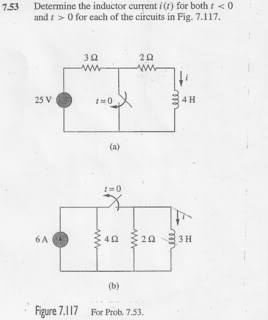# RC Circuits and current

• Engineering
meanswing
This is for question 7.53b) . I understand that the inductor acts like a short circuit but how is the current through the inductor affected? Wont the current source (6 A) split at the node before the 4 ohm resistor and before going into the 2 ohm resistor?

Also to get the Equivalent Resistor when the switch is open is troubling me? At first i wanted to add the 4ohm and 2ohm resistor since they are in series but my answer was wrong. The correct answer was 2ohm? Isnt the 4ohm and 2ohm resistor in series even though the switch is open?viscousflow
For the equivalent resistance, do not forget to zero the current source, when you do, you will immediately see that the 4 Ohm resistor will not be connected to the 2 Ohm resistor in any way.

Also to the first question, when short circuiting the inductor, always think about the path of least resistance, this is the route the current will take.

jimmy neutron
Not sure when this question was posed. Even if it were sometime ago perhaps you may find my comments useful?

With regards Fig 7.53 (b). For times t < 0 when the switch is closed, the inductor acts like a piece of wire (since the current is constant so di/dt = 0 there is no emf induced, VL = L di/dt = 0) and all the 6 A flows through the inductor with no current flow through either the 4 ohm or 2 ohm resistors.

When the swich is opened, only the 4 ohm resistor is in series with the 6 A current source, and all the 6 A flows through this resistor (the current jumps instantaneously from zero to 6 A since a pure resistive circuit).

The inductor and the 2 ohm resistor form a separate circuit, with the inductor connected in series with this resistor. With no source of emf (or constant current sources) in this circuit the current must eventually fall to zero, but this cannot occur instanteously since the inductor inhibits changes in current(instantaneous implies di non-zero for dt = zero).

If the current flowing through this circuit is i(t) then (according to Kirchhoff) the p.d. across the 2 ohm resistor (2*i(t)) is equal to the p.d. across the inductor (3*di/dt).

The polarity of these terms must be considered but I guess that you have
2*i(t) + 3*di/dt = 0.
When looking at differential equations you have to take the boundary conditions into account and this is given by the current through the inductor just before the switch is opened, i.e. i(t=0) = 6 A.

I think this gives the current through the inductor after the switch is opened as something like i(t) = 6*( 1 - exp(-t/tau) ) A where tau is the time constant?

Is this OK? It doesn't look as if the 4 ohm resistor comes into the result for i(t)? Jim

jimmy neutron
Ooops ... the current may be 6 exp(-t/tau) A